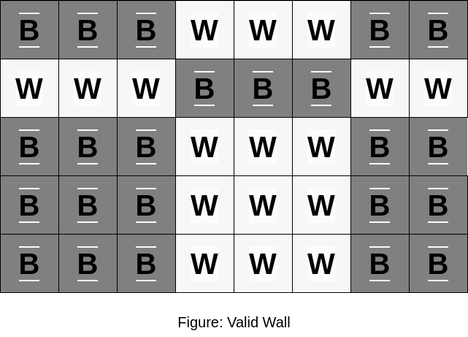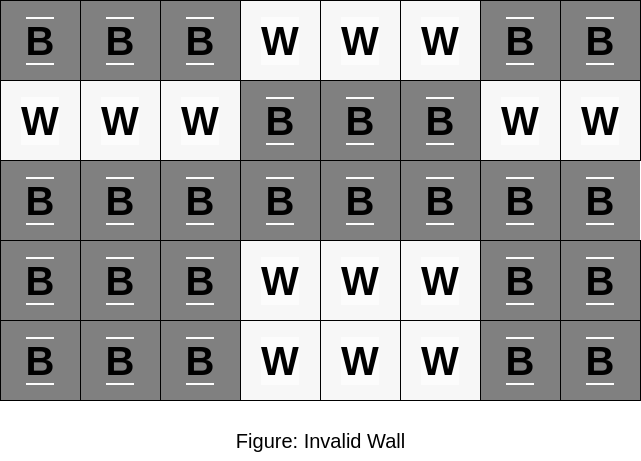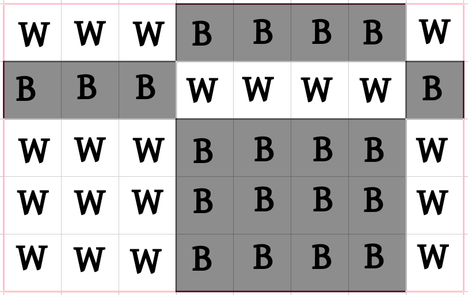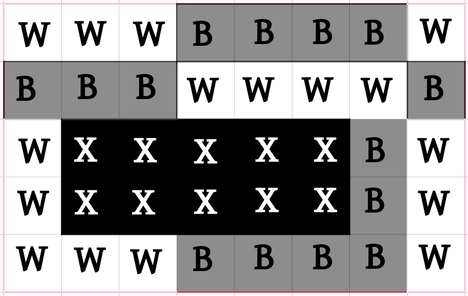# Broken WallborderAmra shobai Raja20/25/74
Limits 1s, 512 MB

Alice has built a rectangular wall of $N \times M$ squares. The wall is made entirely of rectangular black and white bricks.

Every brick is rectangular and consists of some $W \times H$ squares. Note that, all bricks may not be of the same size.

The wall satisfies the following conditions:

• Each brick is either a black brick or a white brick.

• Every square in a black brick is colored black(‘B’) and every square in a white brick is colored white(‘W’).

• Two bricks sharing a side have different colors. Two bricks are either horizontally adjacent or vertically adjacent.

• If two bricks share a side, then the side they share is of equal length i.e., if two bricks are horizontally adjacent, then their height $H$ is the same. And if they are vertically adjacent, then their width $W$ is the same.Bob is Alice’s archenemy. His mission in life is to destroy everything Alice loves. This time, he managed to destroy some part of the wall (maybe even all of it). He created a rectangular hole of size $A \times B$ in Alice’s wall.

Alice has come to you asking for help to reconstruct her wall. During reconstruction, you can only change the squares in which Bob put a hole to either black or white squares. Note that, you cannot change the color of a square that isn’t destroyed.

All she can tell you is how the wall looks right now after Bob put a hole in it. You have to print how the wall looked before Bob destroyed it. If there are multiple valid solutions you can output any of them.

## Input

First line of input contains an integer $T(1 \leq T \leq 10)$— the number of test cases.

First line of each test case contains two integers $N(1 \leq N \leq 10)$ and $M(1 \leq M \leq 10)$— dimensions of the wall.

Each of the following $N$ lines contains $M$ characters representing the wall. ‘B’ represents a black-colored square, ‘W’ represents a white-colored square and ‘X’ represents a hole.

NB: It is guaranteed that $1\le A \le N$ and $1\le B \le M$.

## Output

For each test case, output $N$ lines each containing $M$ characters that represent the wall before Bob put a hole in it. If there are multiple valid ways to reconstruct the wall, you can output any of them.

## Samples

InputOutput
1
5 8
WWWBBBBW
BBBWWWWB
WXXXXXBW
WXXXXXBW
WWWBBBBW

WWWBBBBW
BBBWWWWB
WWWBBBBW
WWWBBBBW
WWWBBBBW


For the first case,

the wall before Bob put a hole in it:The wall after Bob put a hole in it:InputOutput
2
4 4
XXXX
XXXX
WWWW
BBBB
2 3
XXB
XXW

BBBB
BBBB
WWWW
BBBB
BBB
WWW


### Submit